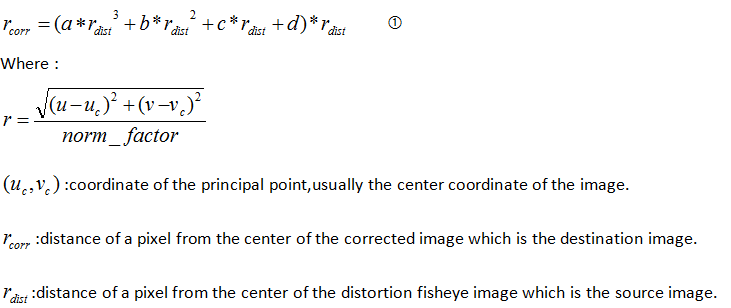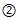Media (Intel® oneAPI Video Processing Library, Intel Media SDK)
Access community support with transcoding, decoding, and encoding in applications using media tools like Intel® oneAPI Video Processing Library and Intel® Media SDK
Announcements
3001 Discussions

## Intel MediaSDK Lens distortion correction (using RAW accelerator) can’t get the same corrected image as PTGUI and MatlabBeginner
233 Views

Intel MediaSDK Lens distortion correction (using RAW accelerator) can’t get the same corrected image as PTGUI and Matlab,even using the same parameters: a b c d.

1.The meida_raw_accelerator_man.pdf describes the 3rd degree polynomial for correcting lens distortion :After doing some research and reading articles,we found the polynomial for correcting lens distortion should be:2.We use polynomialas the correcting polynomial.

Set parameters value: a=0.0925 b=-0.2676 c=-0.0997c=1.2755

Source image: circle.bmpPTGUI result: result_ptgui.bmpMatlab result:result_matlab.bmpMediaSDK result: result_mediasdk_0.bmpMatlab code:untitle.m

```close all; clear all; clc;

tic;
img_gray = rgb2gray(A);
[m,n,k] = size(A);
P =[0.0925   -0.2676   -0.0997    1.2755];
C=[];

x0 = m/2;
y0 = n/2;
for u=1:m
for v=1:n
r = sqrt((u-x0)^2+(v-y0)^2);
rt = polyval(P,r/x0)*r;

if r ==0
C(u,v,1)=A(x0,y0,1);
C(u,v,2)=A(x0,y0,2);
C(u,v,3)=A(x0,y0,3);
else
i = fix((u-x0)*rt/r)+x0;
j = fix((v-y0)*rt/r)+y0;
C(u,v,1)=A(i,j,1);
C(u,v,2)=A(i,j,2);
C(u,v,3)=A(i,j,3);
end
end
end
C = uint8(C);
imshow(C);

toc;```

MediaSDK program: sample_camera_d.exe

MediaSDK command line:

sample_camera_d.exe -i bayer_circle.raw -f bggr -b 16 -w 4000 -h 3000 -lens 0.0925 -0.2676 -0.0997 1.2755 -o result_mediasdk_

PTGUI and Matlab can get the same result with the same params a,b,c and d. But the MediaSDK lens correction fails to get the same result under the same a b c d.

Now ,we call parameters of PTGUI and Matlab as a,b,c,d. The params of MediaSDK are abcdm.we want to know that :

(1)we know the value of the params a b c and d, how to set the value of abcdto get the same corrected image as PTGUI and Matlab.

(2)What’s the relationship between a b c d and  abcdm

(3)Does mediaSDK use polynomial? If not,what is the mediaSDK polynomial for correcting.And how can we translate a b c d for quick correcting.

ps.does not the sample_camera.exe support the argb16 input format? the problem is described in the topic:

https://software.intel.com/en-us/forums/intel-media-sdk/topic/704495